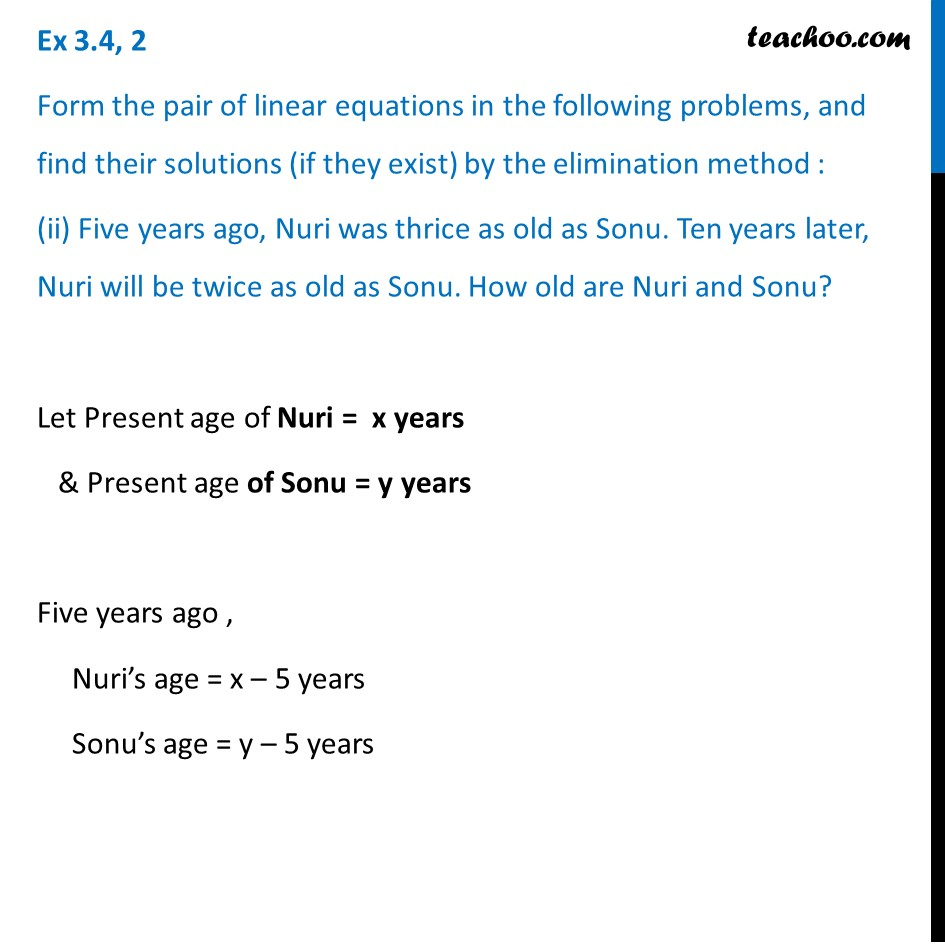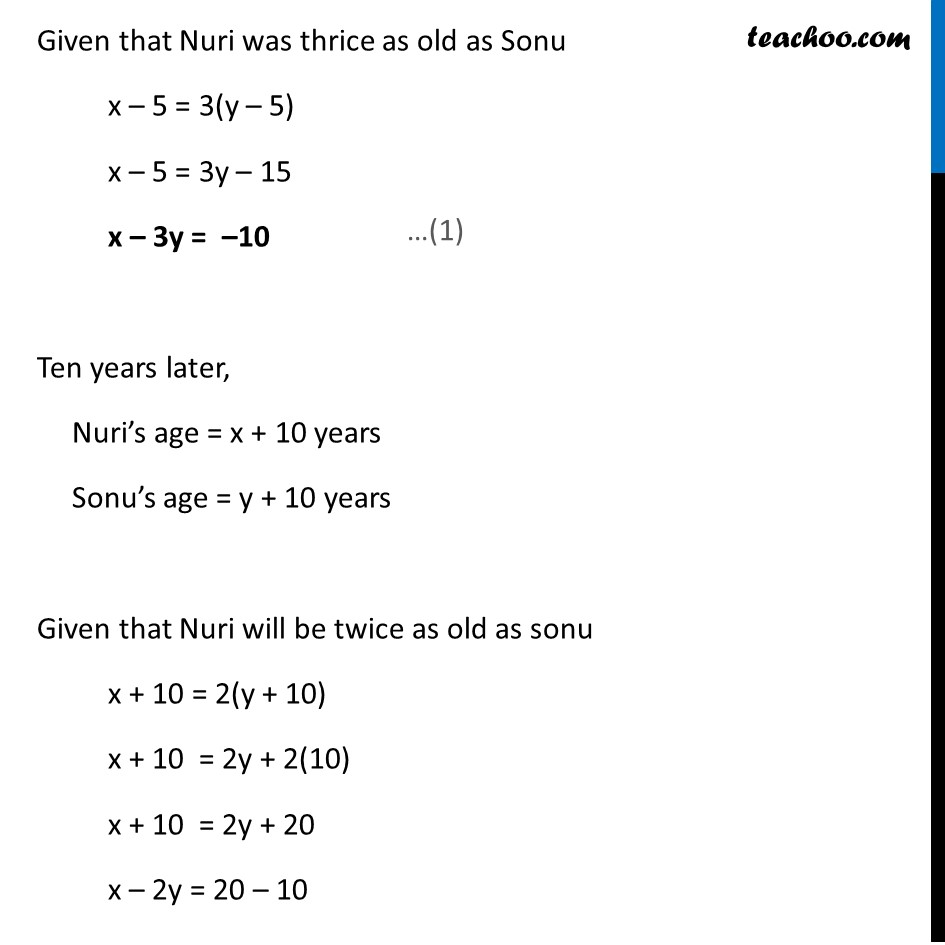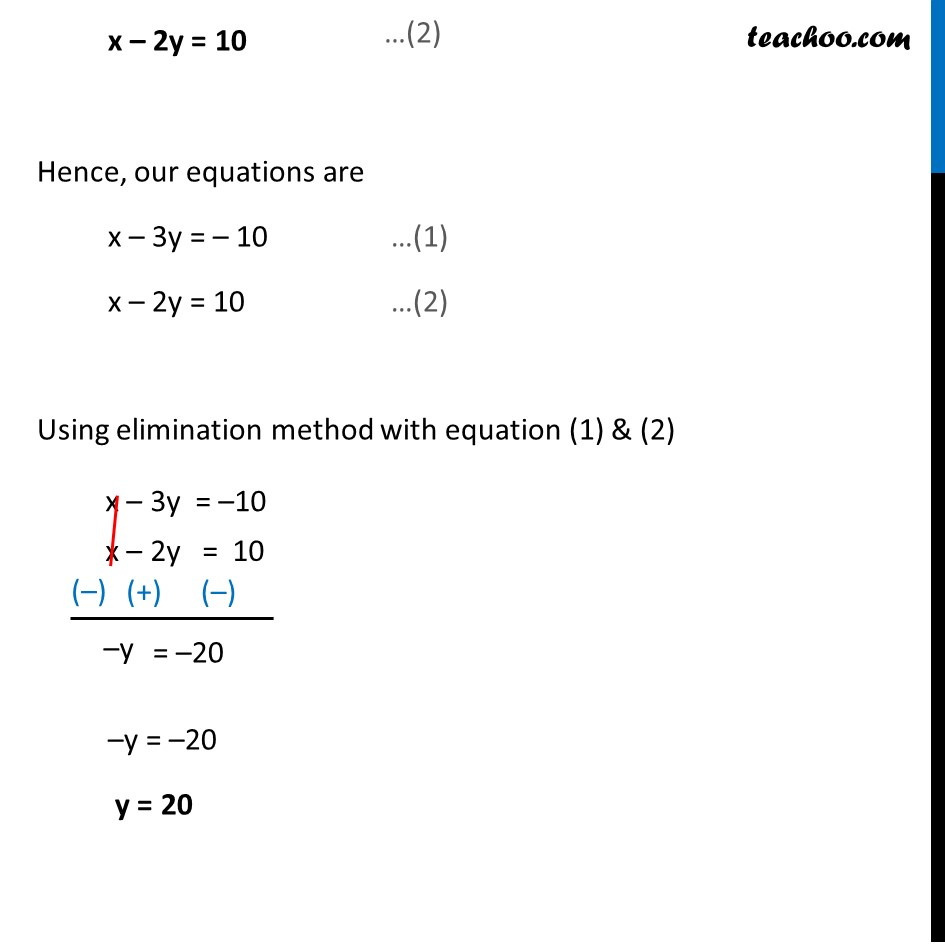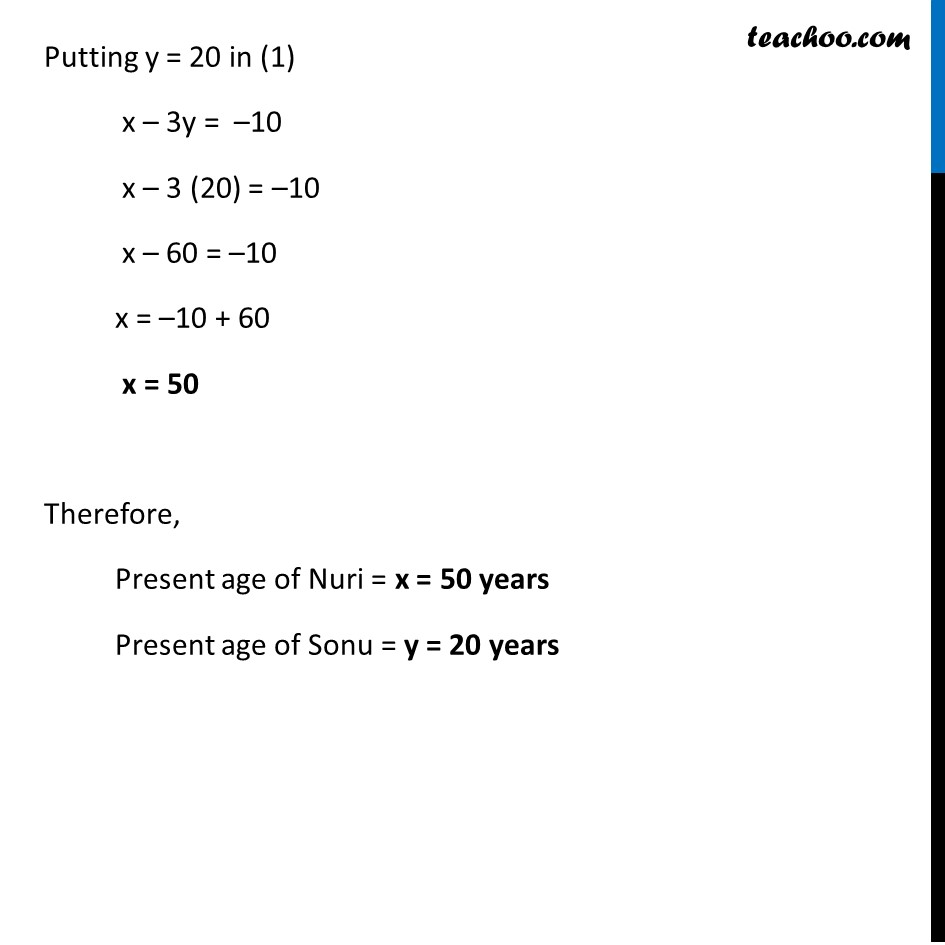Ex 3.4

Chapter 3 Class 10 Pair of Linear Equations in Two Variables (Term 1)
Serial order wise### Transcript

Ex 3.4, 2 Form the pair of linear equations in the following problems, and find their solutions (if they exist) by the elimination method : (ii) Five years ago, Nuri was thrice as old as Sonu. Ten years later, Nuri will be twice as old as Sonu. How old are Nuri and Sonu? Let Present age of Nuri = x years & Present age of Sonu = y years Five years ago , Nuri’s age = x – 5 years Sonu’s age = y – 5 years Given that Nuri was thrice as old as Sonu x – 5 = 3(y – 5) x – 5 = 3y – 15 x – 3y = –10 Ten years later, Nuri’s age = x + 10 years Sonu’s age = y + 10 years Given that Nuri will be twice as old as sonu x + 10 = 2(y + 10) x + 10 = 2y + 2(10) x + 10 = 2y + 20 x – 2y = 20 – 10 x – 2y = 10 Hence, our equations are x – 3y = – 10 …(1) x – 2y = 10 …(2) Using elimination method with equation (1) & (2) –y = –20 y = 20 Putting y = 20 in (1) x – 3y = –10 x – 3 (20) = –10 x – 60 = –10 x = –10 + 60 x = 50 Therefore, Present age of Nuri = x = 50 years Present age of Sonu = y = 20 years